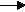gcsescience.com                                       6                                       gcsescience.com

Rates of Reaction

How can the Rate
of the Decomposition of Hydrogen Peroxide be Measured?

Hydrogen peroxide decomposes to make oxygen gas and water.
The rate of this reaction is very slow unless a catalyst is used.

hydrogen peroxideoxygen   +   water.
2H2O2(aq)O2(g)   +   2H2O(l)

The rate of this reaction can be measured by looking
at the rate at which the product oxygen gas is formed.

The reaction is carried out in a closed flask which has
a gas syringe connected to the top of it. The reaction is
started by adding a catalyst to the hydrogen peroxide
solution
. The catalyst used is often manganese(IV) oxide.

The volume of oxygen in the syringe increases as the
reaction proceeds. The volume of oxygen can be recorded
every 30 seconds by reading the marks on the syringe
and then a graph of volume against time can be plotted.
The gradient of the plot (the steepness of the slope)
shows the rate of the decomposition (how fast it is going).

gcsescience.com      The Periodic Table      Index      Reaction Rate Quiz      gcsescience.com